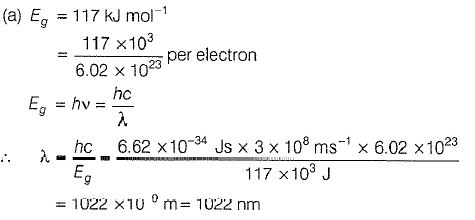Courses

# Revisal Problems (Past 13 Year) JEE Main (Solid State)

## 18 Questions MCQ Test Chemistry for JEE Advanced | Revisal Problems (Past 13 Year) JEE Main (Solid State)

Description
This mock test of Revisal Problems (Past 13 Year) JEE Main (Solid State) for JEE helps you for every JEE entrance exam. This contains 18 Multiple Choice Questions for JEE Revisal Problems (Past 13 Year) JEE Main (Solid State) (mcq) to study with solutions a complete question bank. The solved questions answers in this Revisal Problems (Past 13 Year) JEE Main (Solid State) quiz give you a good mix of easy questions and tough questions. JEE students definitely take this Revisal Problems (Past 13 Year) JEE Main (Solid State) exercise for a better result in the exam. You can find other Revisal Problems (Past 13 Year) JEE Main (Solid State) extra questions, long questions & short questions for JEE on EduRev as well by searching above.
QUESTION: 1

### Only One Option Correct Type This section contains 18 multiple choice questions. Each question has four choices (a), (b), (c) and (d), out of which ONLY ONE is correct.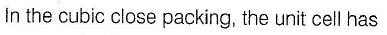Solution: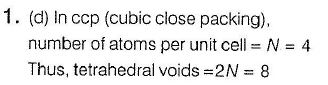QUESTION: 2

###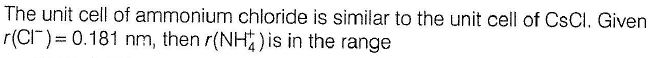Solution: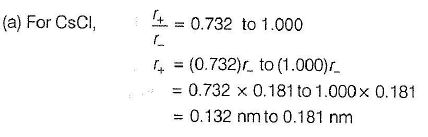QUESTION: 3

###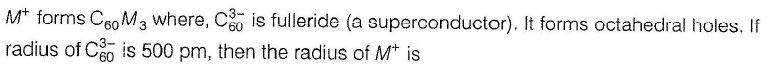Solution: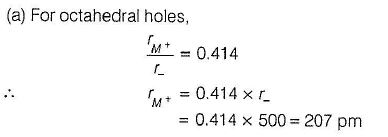QUESTION: 4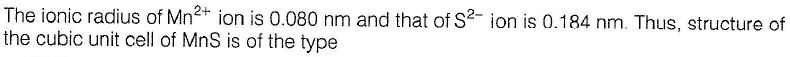Solution: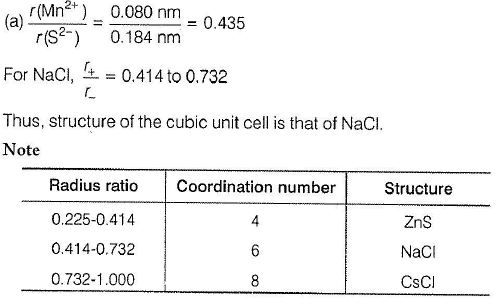QUESTION: 5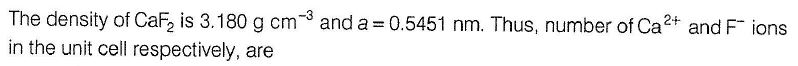Solution: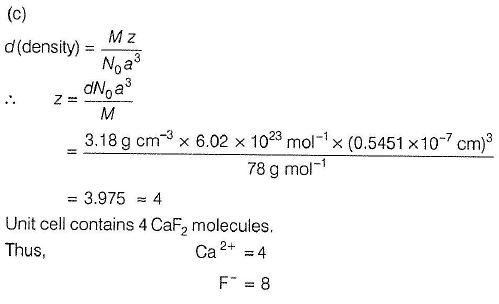QUESTION: 6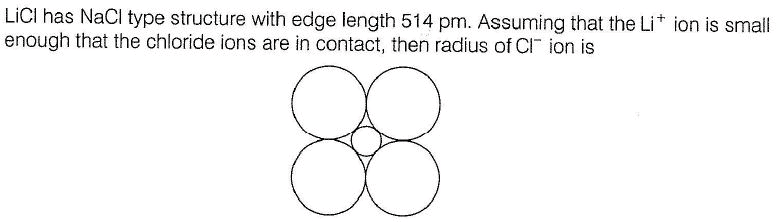Solution: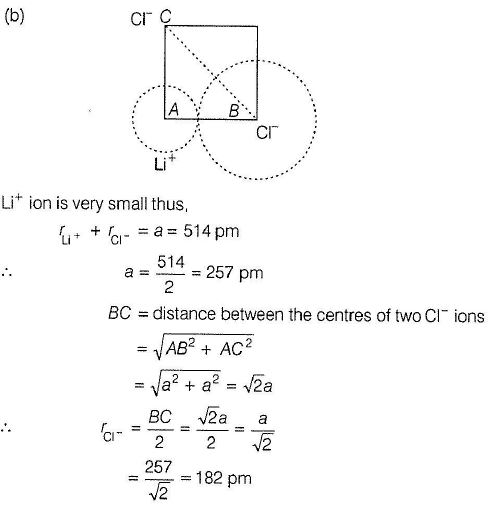QUESTION: 7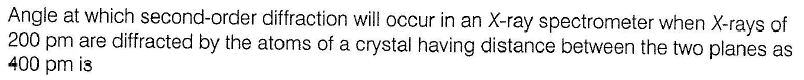Solution: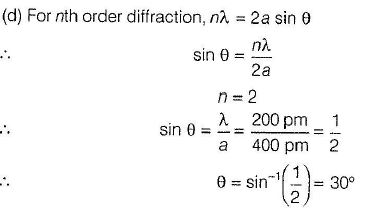QUESTION: 8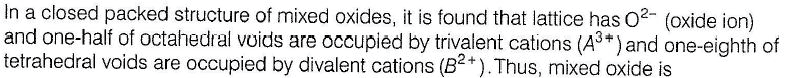Solution: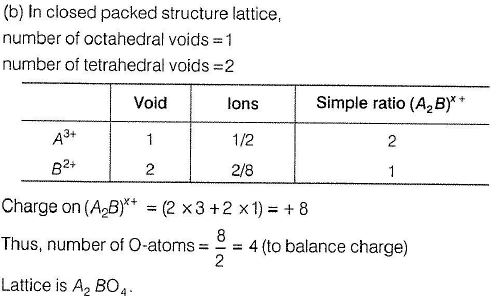QUESTION: 9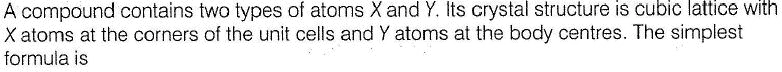Solution: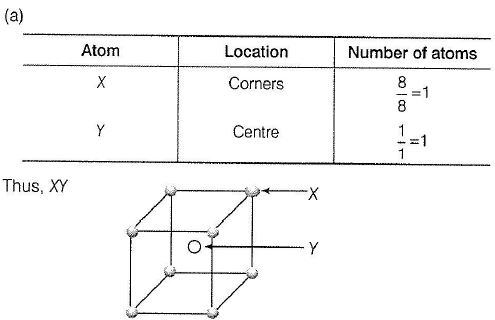QUESTION: 10

A compound is formed by two elements M and N. The element N forms ccp and atoms of M occupy 1/3rd of tetrahedral voids. What is the formula of the compound?

Solution:
QUESTION: 11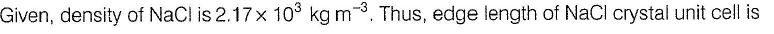Solution: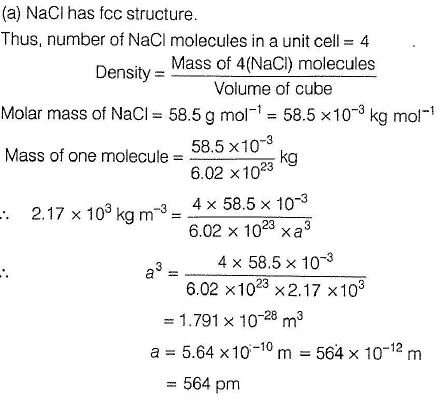QUESTION: 12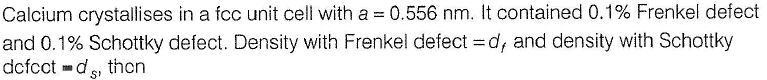Solution: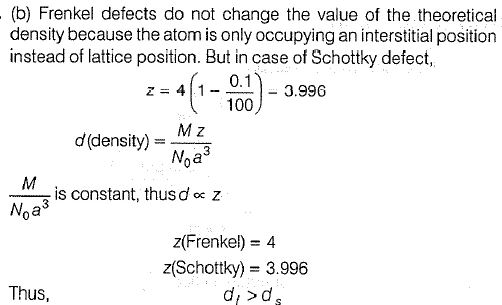QUESTION: 13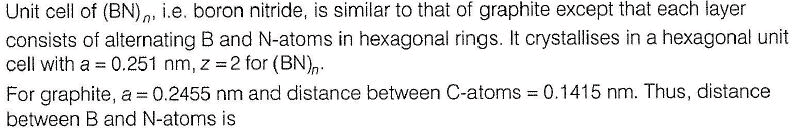Solution: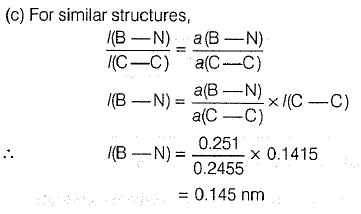QUESTION: 14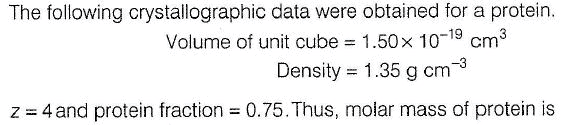Solution: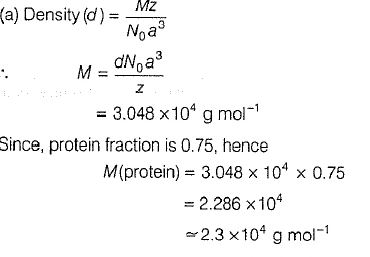QUESTION: 15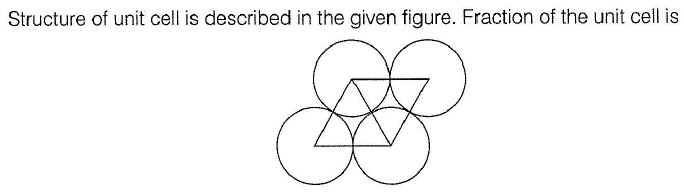Solution: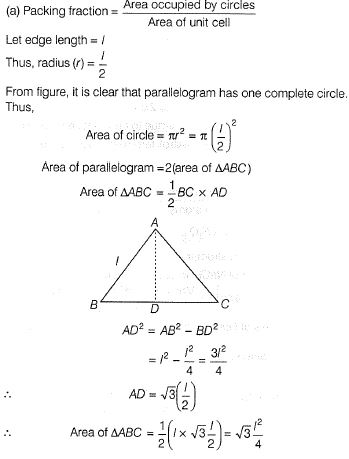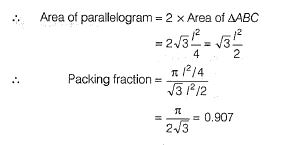QUESTION: 16

CsCl crystallises in body centred cubic lattice. If 'a' is its edge length then which of the following expressions is correct ?

Solution:
QUESTION: 17

How many unit cells are present in a cube-shaped ideal crystal of NaCl of mass 1.0 g?

Solution: Molar mass of Na = 23 and Cl = 35.5 So , the molecular mass of NaCl is 23 + 35.5 =58.5 . NaCl occupies fcc structure i.e 4 atoms will constitute 1 unit cell. Avagadro’s no = 6.023 × 10 ^ 23 number of unit cell = 6.023 × 10^23 /58.5*4 = 6.023 × 10 ^ 23 / 234 = 2.57 × 10^ 21
QUESTION: 18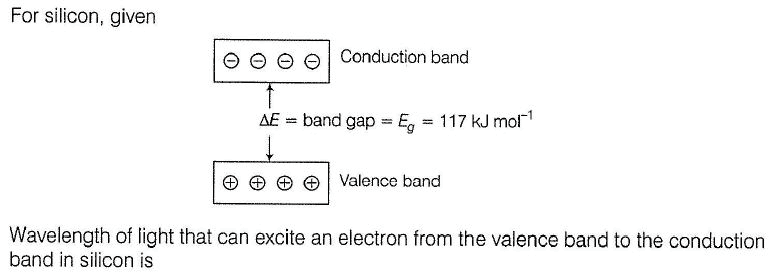Solution: